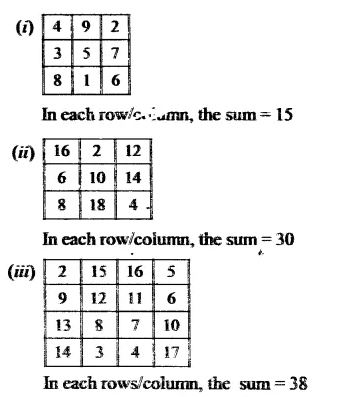# RS Aggarwal Solutions Class 6 Chapter 3 Whole Numbers Exercise 3B

In this chapter, we provide RS Aggarwal Solutions for Class 6 Chapter 3 Whole Numbers Exercise 3B for English medium students, Which will very helpful for every student in their exams. Students can download the latest RS Aggarwal Solutions for Class 6 Chapter 3 Whole Numbers Exercise 3B pdf, free RS Aggarwal Solutions Class 6 Chapter 3 Whole Numbers Exercise 3B book pdf download. Now you will get step by step solution to each question.

### RS Aggarwal Class 6 Chapter 3 Whole Numbers Ex 3B Download PDF

Question 1.
Solution:
(i) 458 + 639 = 639 + 458 (Commulative law)
(ii) 864 + 2006 = 2006 + 864 (Commulative law)
(iii) 1946 + 984 = 984 + 1946 (Commulative law)
(iv) 8063 + 0 = 8063 (Additive property of zero)
(v) 53501 + (574 + 799) = 574 + (53501 + 799) (Associative law)

Question 2.
Solution:
(i) 16509 + 114 = 16623
Check : 16623 – 114 = 16509 which is given.
(ii) 2359 + 548 = 2907
Check: 2907 – 2359 = 548 which is given
(iii) 19753 + 2867 = 22620
Check : 22620 – 19753 = 2867 which is given

Question 3.
Solution:
(1546 + 498) + 3589
= 2044 + 3589
= 5633
and 1546 + (498 + 3589)
= 1546 + 4087
= 5633
Yes, the above two sum are equal.
The property used is associative law of addition.

Question 4.
Solution:
(i) 953 + 707 + 647
= (953 + 647) + 707
(by associative law)
= 1600 + 707
= 2307
(ii) 1983 + 647 + 217 + 353
= (1983 + 217) + (647 + 353)
= 2200 + 1000 = 3200
(iii) 15409 + 278 + 691 + 422
= (15409 + 691) + (278 + 422)
(by associative law)
= 16100 + 700
= 16800
(iv) 3259 + 10001 + 2641 + 9999
= (3259 + 2641) + (10001 + 9999)
(by associative law)
= 5900 + 20000
= 25900
(v) 1 +2 + 3 +4 + 96 + 97 + 98 + 99
= (1 +99) + (2 + 98) + (3 + 97) + (4 + 96)
= (100+ 100) + (100 +100)
= 200 + 200
= 400
(vi) 2 + 3 + 4 + 5 + 45 + 46 + 47 + 48
= (2 + 48) + (3 + 47) + (4 + 46) + (5 + 45)
= (50 + 50) + (50 + 50)
= 100 + 100 = 209

Question 5.
Solution:
(i) 6784 + 9999 = (6784 – 1) + (9999 + 1)
= 6783 + 10000
= 16783
(ii) 10578 + 99999
= (10578 – 1) + (99999 + 1)
= 10577 + 100000
= 110577

Question 6.
Solution:
Yes it is true, by the property of associative law of addition.

Question 7.
Solution:
The magic squares given are completed as under:In each row/column, the sum = 46

Question 8.
Solution:
(i) The sum of two odd numbers is an odd number (F)
As sum of two odd numbers is alway an even number
(ii) The sum of two even number is an even number (T)
(iii) The sum of an even number and an odd number is an odd number (T)

All Chapter RS Aggarwal Solutions For Class 6 Maths

—————————————————————————–

All Subject NCERT Exemplar Problems Solutions For Class 6

All Subject NCERT Solutions For Class 6

*************************************************

I think you got complete solutions for this chapter. If You have any queries regarding this chapter, please comment on the below section our subject teacher will answer you. We tried our best to give complete solutions so you got good marks in your exam.

If these solutions have helped you, you can also share rsaggarwalsolutions.in to your friends.